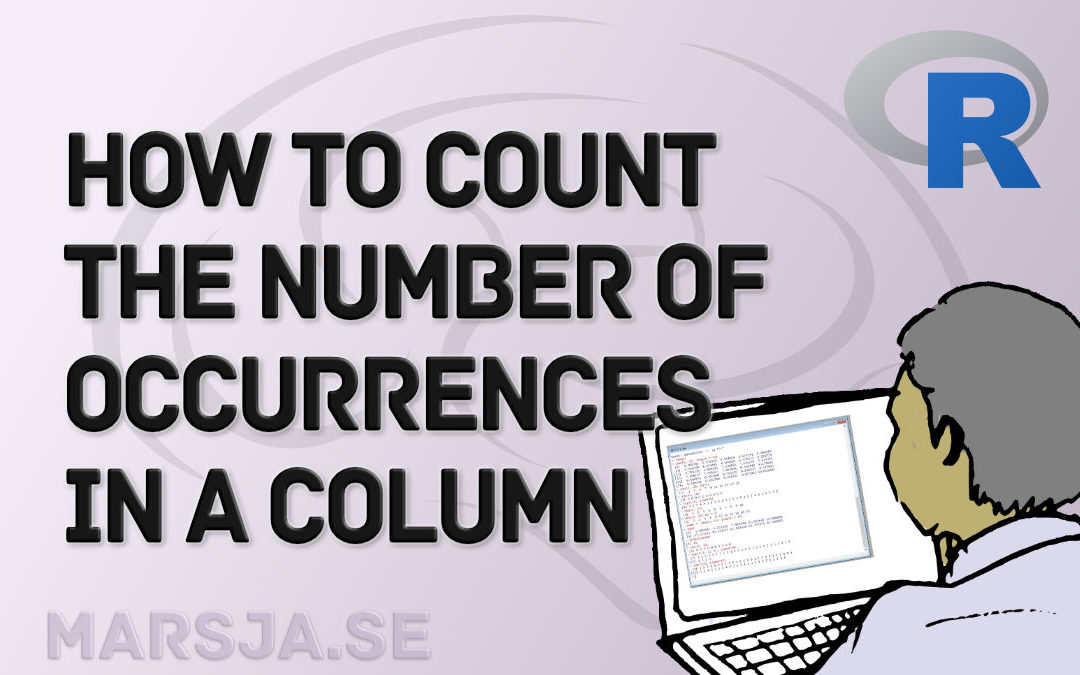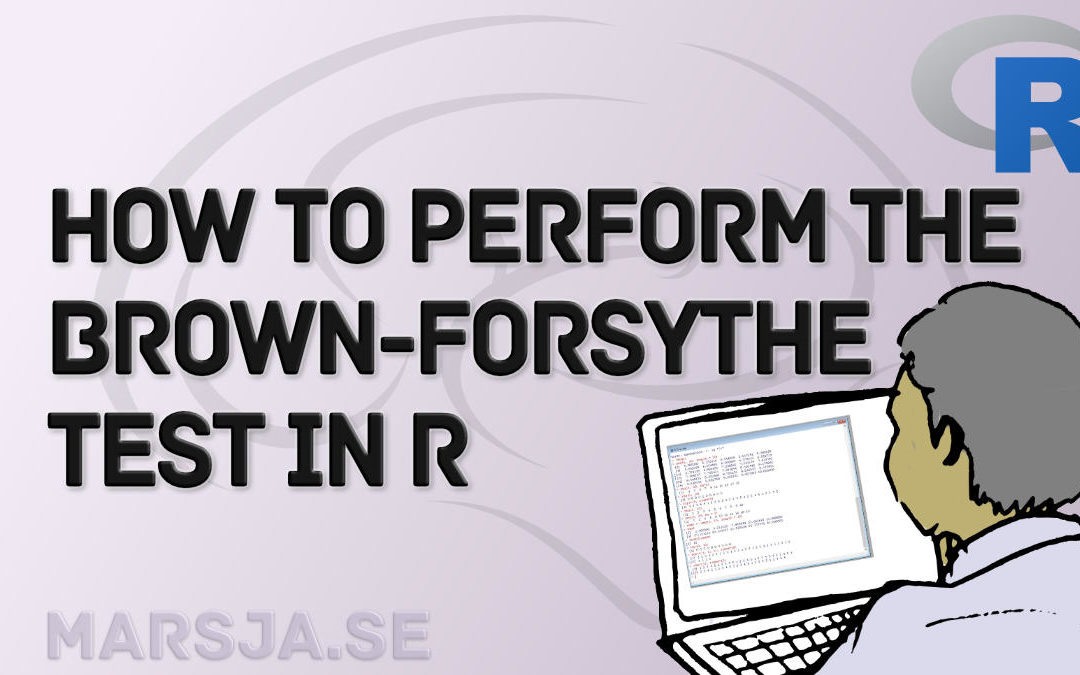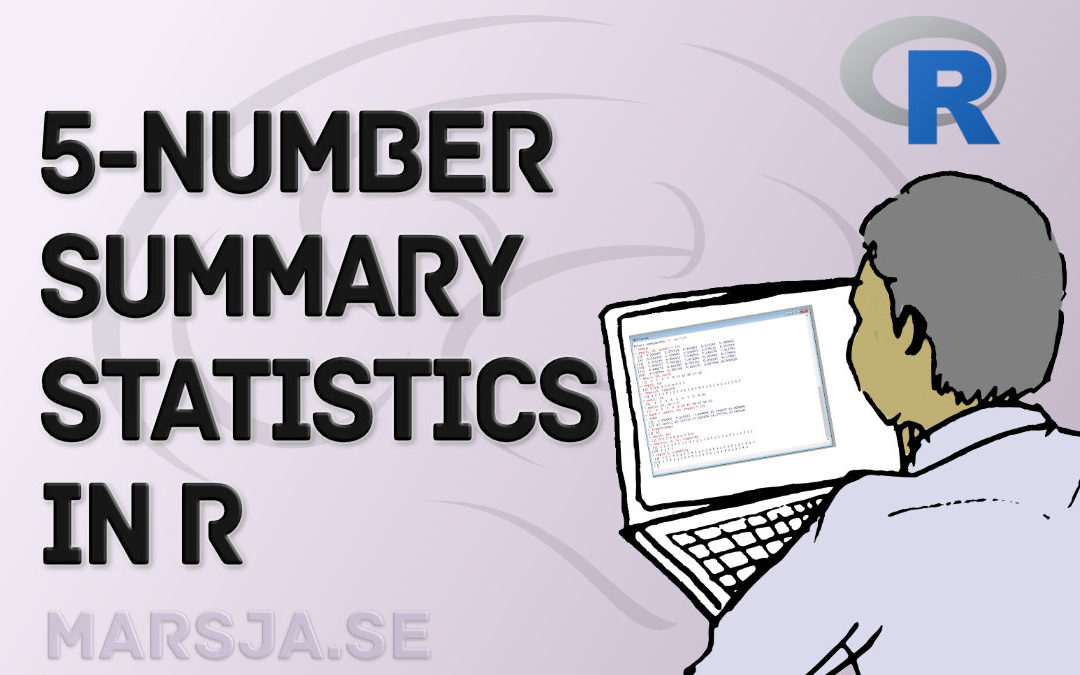## Learn How to Convert Matrix to dataframe in R with base functions & tibble

In this short tutorial, you will learn how to convert a matrix to a dataframe in R. Specifically, you will learn how to use base R and the package tibble to change the matrix to a dataframe. You will learn this task by 4 different examples (2 using each method). ...## R Count the Number of Occurrences in a Column using dplyr

In this R tutorial, you are going to learn how to count the number of occurrences in a column. Sometimes, before starting to analyze your data,  it may be useful to know how many times a given value occurs in your variables. For example, when you have a limited...## How to Do the Brown-Forsythe Test in R: A Step-By-Step Example

In this tutorial, you will learn how to do the Brown-Forsythe test in R. This test is great as you can use it to test the assumption of homogeneity of variances, which is important for e.g. Analysis of Variance (ANOVA).  Outline of the Post This post is...## How to Concatenate Two Columns (or More) in R – stringr, tidyr

In this guide you will learn how to concatenate two columns in R. In fact, you will learn how to merge multiple columns in R using base R (e.g., using the paste function) and Tidyverse (e.g. using str_c() and unite()). In the final section of this post, you will learn...## How to Calculate Five-Number Summary Statistics in R

In this short tutorial, you will learn how to find the five-number summary statistics in R. Specifically, in this post we will calculate: MinimumLower-hingeMedianUpper-hingeMaximum Now, we will also visualize the five-number summary statistics using a boxplot. First,...